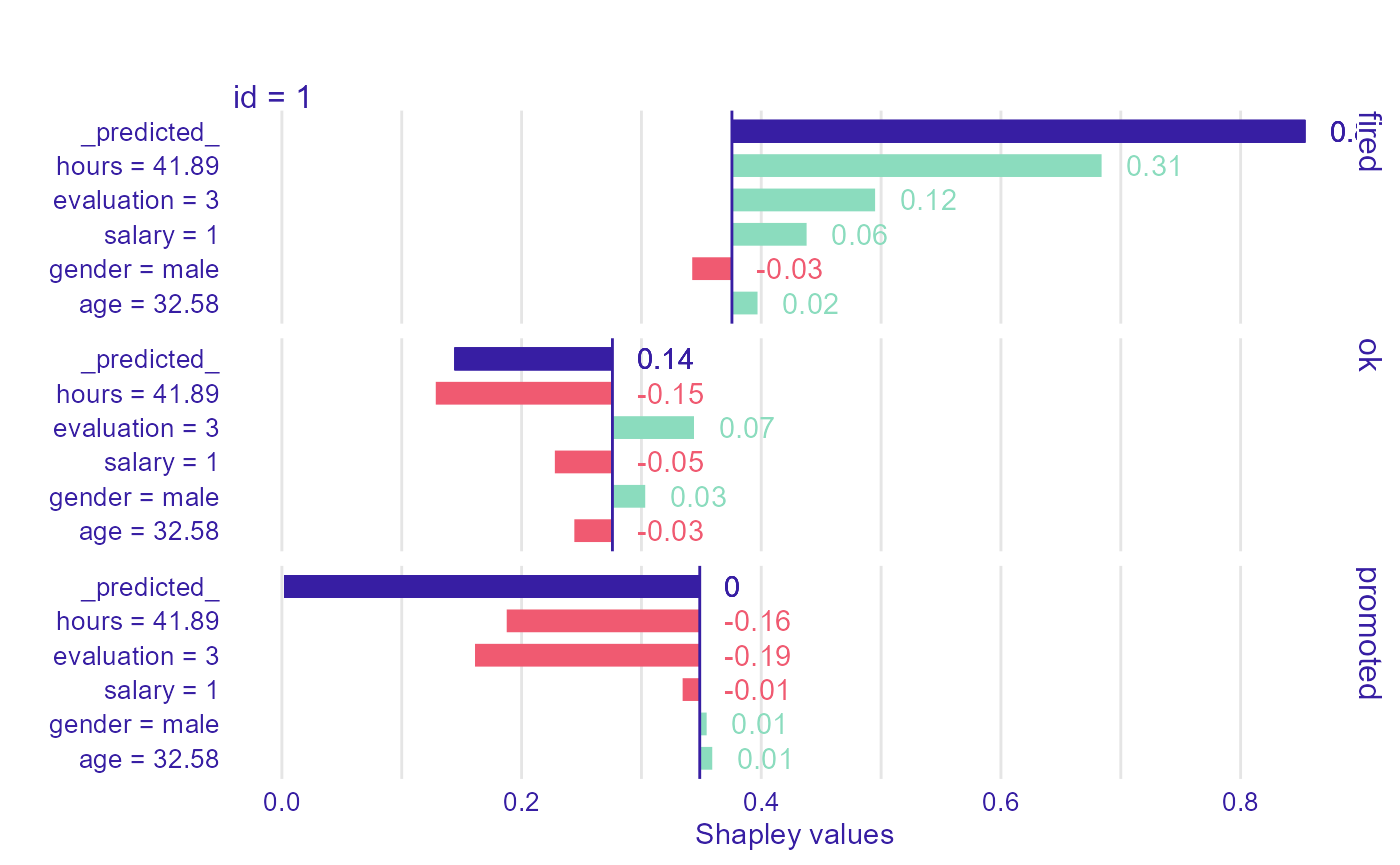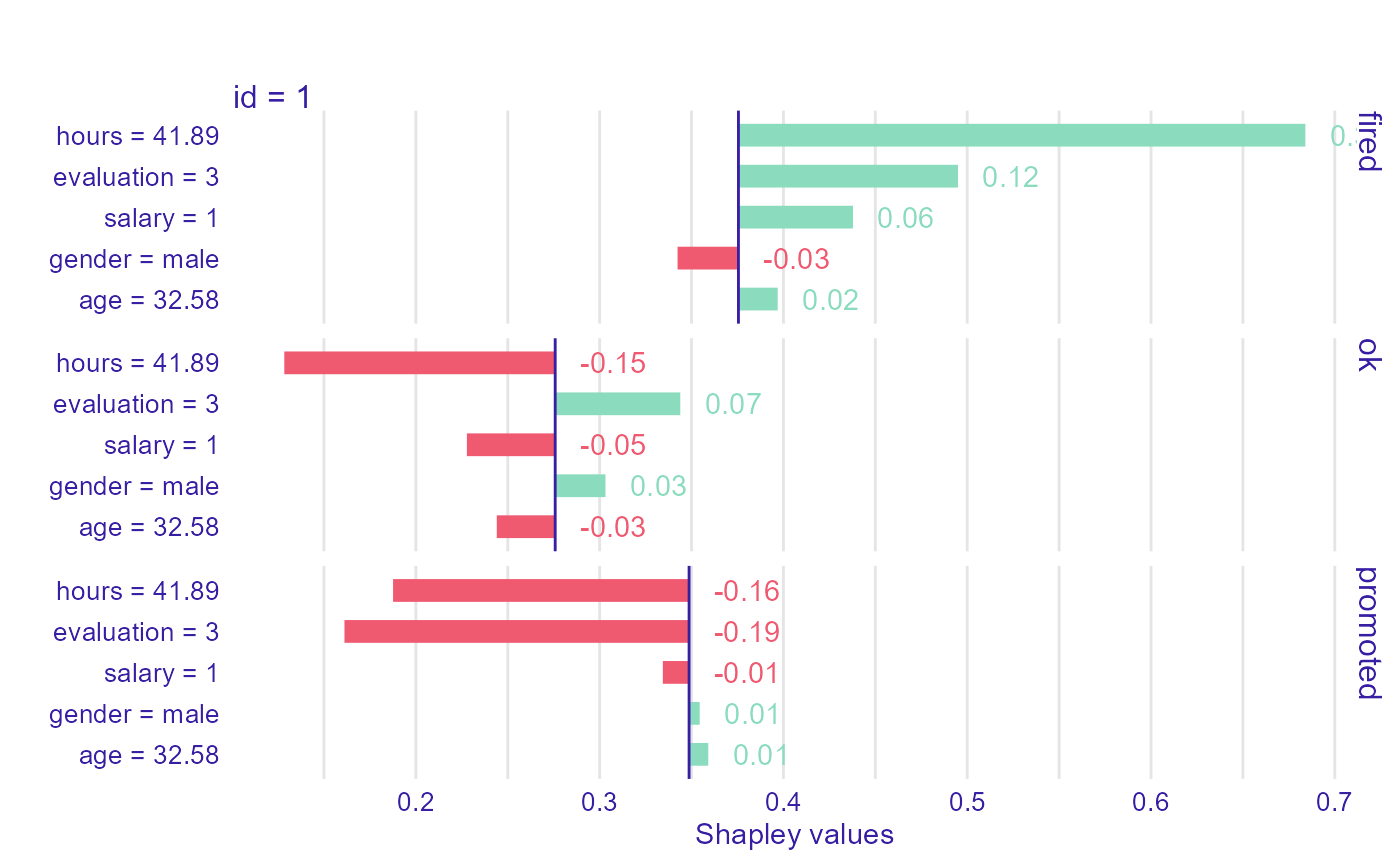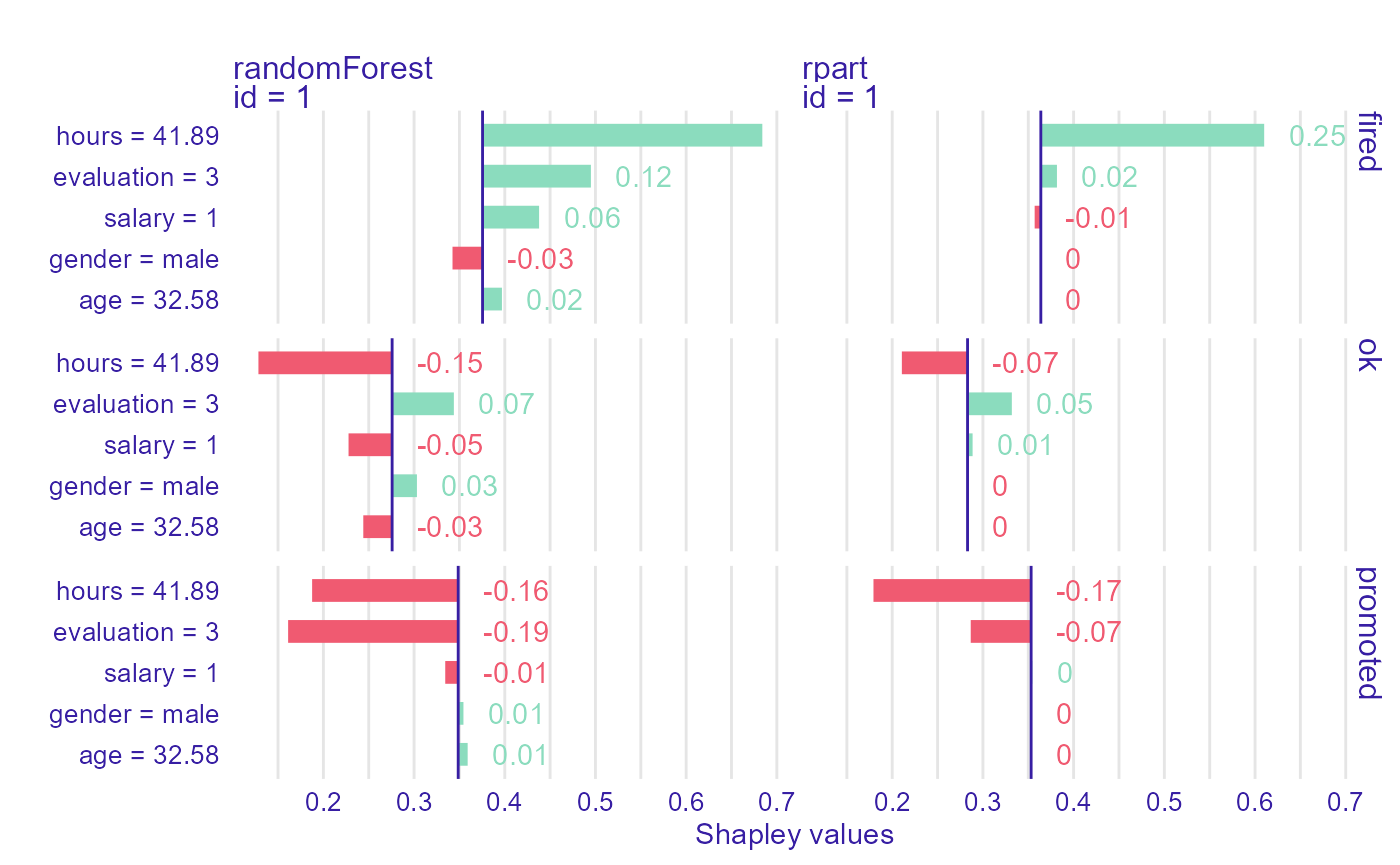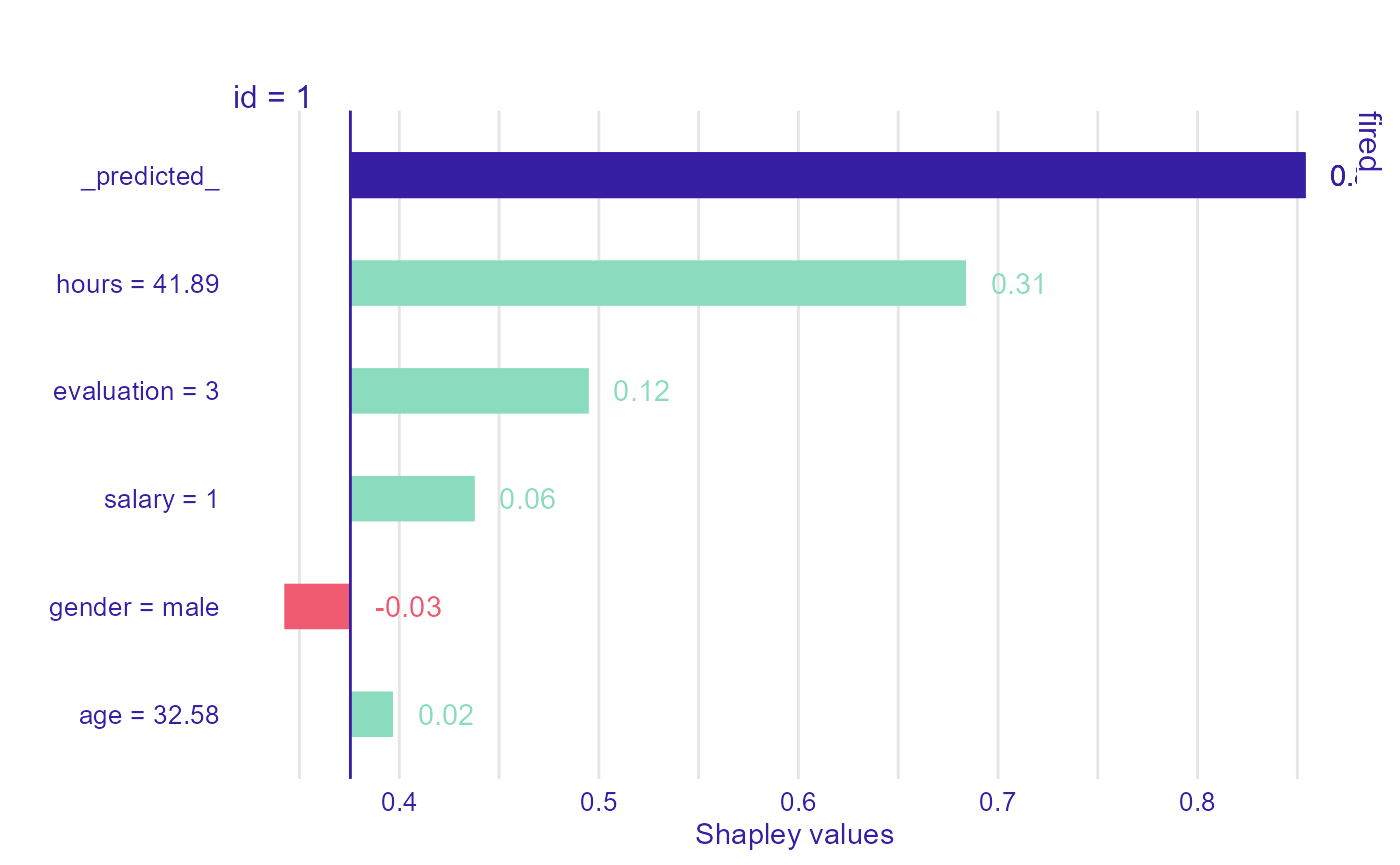# Introduction

The shapper is an R package which ports the shap python library in R. For details and examples see shapper repository on github and shapper website.

SHAP (SHapley Additive exPlanations) is a method to explain predictions of any machine learning model. For more details about this method see shap repository on github.

## Python library shap

To run shapper python library shap is required. It can be installed both by python or R. To install it throught R, you an use function install_shap from the shapper package.

shapper::install_shap()

The example usage is presented on the HR dataset from the R package DALEX. For more details see DALEX github repository.
library("DALEX")
Y_train <- HR$status x_train <- HR[ , -6] # Let’s build models library("randomForest") set.seed(123) model_rf <- randomForest(x = x_train, y = Y_train) library(rpart) model_tree <- rpart(status~. , data = HR) # Here shapper starts First step is to create an explainer for each model. The explainer is an object that wraps up a model and meta-data. library(shapper) p_function <- function(model, data) predict(model, newdata = data, type = "prob") ive_rf <- individual_variable_effect(model_rf, data = x_train, predict_function = p_function, new_observation = x_train[1:2,], nsamples = 50) ive_tree <- individual_variable_effect(model_tree, data = x_train, predict_function = p_function, new_observation = x_train[1:2,], nsamples = 50) ive_rf ## gender age hours evaluation salary _id_ _ylevel_ _yhat_ _yhat_mean_ ## 1 male 32.58267 41.88626 3 1 1 fired 0.854 0.3755216 ## 1.3 male 32.58267 41.88626 3 1 1 fired 0.854 0.3755216 ## 1.4 male 32.58267 41.88626 3 1 1 fired 0.854 0.3755216 ## 1.5 male 32.58267 41.88626 3 1 1 fired 0.854 0.3755216 ## 1.6 male 32.58267 41.88626 3 1 1 fired 0.854 0.3755216 ## 1.1 male 32.58267 41.88626 3 1 1 ok 0.144 0.2758111 ## _vname_ _attribution_ _sign_ _label_ ## 1 gender -0.03312199 - randomForest ## 1.3 age 0.02135603 + randomForest ## 1.4 hours 0.30846492 + randomForest ## 1.5 evaluation 0.11945970 + randomForest ## 1.6 salary 0.06231975 + randomForest ## 1.1 gender 0.02735491 + randomForest # Plotting results plot(ive_rf, bar_width = 4)To see only attributions use option show_predicted = FALSE. plot(ive_rf, show_predicted = FALSE, bar_width = 4)We can show many models on one grid. plot(ive_rf, ive_tree, show_predicted = FALSE, bar_width = 4)## Let’s filter data for plot ive_rf_filtered <- ive_rf[ive_rf$_ylevel_ =="fired", ]
shapper:::plot.individual_variable_effect(ive_rf_filtered)